# Pythagorean theorem - 9th grade (14y) - math problems

#### Number of problems found: 539

• Triangle IRTIn isosceles right triangle ABC with right angle at vertex C is coordinates: A (-1, 2); C (-5, -2) Calculate the length of segment AB.
• Inscribed rectangle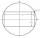The circle area is 216. Determine the area of inscribed rectangle with one side 5 long.
• Sphere cutsAt what distance from the center intersects sphere with radius R = 56 plane, if the cut area and area of the main sphere circle is in ratio 1/2.
• Height of pyramidThe pyramid ABCDV has edge lengths: AB = 4, AV = 7. What is its height?
• Spruce heightHow tall was spruce that was cut at an altitude of 8m above the ground and the top landed at a distance of 15m from the heel of the tree?
• The chordCalculate a chord length which the distance from the center of the circle (S, 6 cm) equals 3 cm.
• Body diagonalCalculate the volume and surface of the cube if the body diagonal measures 10 dm.
• Trapezoid ABCD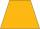Calculate the perimeter of trapezoid ABCD if we know the side c=15, b=19 which is also a height and side d=20.
• Diamond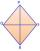Calculate the length of the two diagonals of the diamond if: a = 13 cm v = 12 cm
• Forces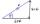Determine the resultant of two perpendicular forces F1 = 560 N and second force of 25% smaller.
• VectorsVector a has coordinates (8; 10) and vector b has coordinates (0; 17). If the vector c = b - a, what is the magnitude of the vector c?
• Find the 3Find the distance and midpoint between A(1,2) and B(5,5).
• VectorCalculate length of the vector v⃗ = (9.75, 6.75, -6.5, -3.75, 2).
• Three pointsThree points A (-3;-5) B (9;-10) and C (2;k) . AB=AC What is value of k?The double ladder shoulders should be 3 meters long. What height will the upper top of the ladder reach if the lower ends are 1.8 meters apart?
• Acreage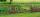What acreage has a rectangular plot whose diagonal is 34 meters long and one side has a length of 16 meters. ?
• WasteHow many percents are waste from a circular plate with a radius of 1 m from which we cut a square with the highest area?
• Diagonal to areaCalculate the area of a rectangle in which the length of the diagonal is 10 cm.
• IsoscelesIsosceles trapezium ABCD ABC = 12 angle ABC = 40 ° b=6. Calculate the circumference and area.
• Square diagonalCalculate the length of the square diagonal if the perimeter is 172 cm.

Do you have an interesting mathematical word problem that you can't solve it? Submit a math problem, and we can try to solve it.

We will send a solution to your e-mail address. Solved examples are also published here. Please enter the e-mail correctly and check whether you don't have a full mailbox.

Please do not submit problems from current active competitions such as Mathematical Olympiad, correspondence seminars etc...

Pythagorean theorem is the base for the right triangle calculator. Pythagorean theorem - math word problems. Examples for 9th grade.